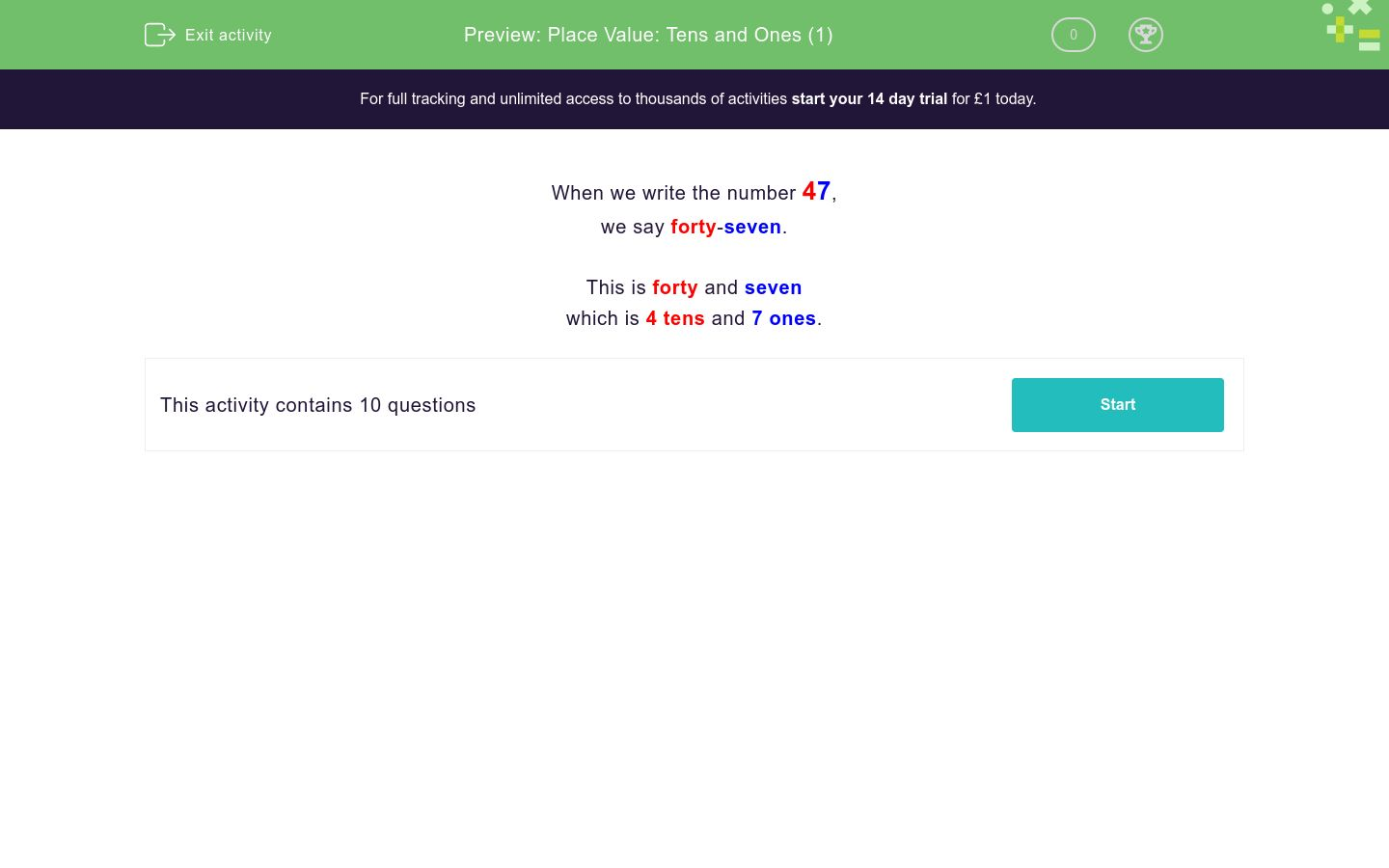# Place Value: Tens and Ones (1)

In this worksheet, students must split two-digit numbers into tens and ones.Key stage:  KS 1

Curriculum topic:   Number: Number and Place Value

Curriculum subtopic:   Recognise Tens and Ones

Difficulty level:### QUESTION 1 of 10

When we write the number 47,

we say forty-seven.

This is forty and seven

which is 4 tens and 7 ones.

In the grid below, show the number:

47

 Tens Ones 4 7

In the grid below, show the number:

74

 Tens Ones 4 7

In the grid below, show the number:

35

 Tens Ones 3 5

In the grid below, show the number:

53

 Tens Ones 3 5

In the grid below, show the number:

61

 Tens Ones 1 6

In the grid below, show the number:

16

 Tens Ones 1 6

In the grid below, show the number:

12

 Tens Ones 1 2

In the grid below, show the number:

21

 Tens Ones 1 2

In the grid below, show the number:

89

 Tens Ones 8 9

In the grid below, show the number:

98

 Tens Ones 8 9
• Question 1

In the grid below, show the number:

47

 Tens Ones 4 7
EDDIE SAYS
4 tens and 7 ones.
• Question 2

In the grid below, show the number:

74

 Tens Ones 4 7
EDDIE SAYS
7 tens and 4 ones.
• Question 3

In the grid below, show the number:

35

 Tens Ones 3 5
EDDIE SAYS
3 tens and 5 ones.
• Question 4

In the grid below, show the number:

53

 Tens Ones 3 5
EDDIE SAYS
5 tens and 3 ones.
• Question 5

In the grid below, show the number:

61

 Tens Ones 1 6
EDDIE SAYS
6 tens and 1 one.
• Question 6

In the grid below, show the number:

16

 Tens Ones 1 6
EDDIE SAYS
1 ten and 6 ones.
• Question 7

In the grid below, show the number:

12

 Tens Ones 1 2
EDDIE SAYS
1 ten and 2 ones.
• Question 8

In the grid below, show the number:

21

 Tens Ones 1 2
EDDIE SAYS
2 tens and 1 one.
• Question 9

In the grid below, show the number:

89

 Tens Ones 8 9
EDDIE SAYS
8 tens and 9 ones.
• Question 10

In the grid below, show the number:

98

 Tens Ones 8 9
EDDIE SAYS
9 tens and 8 ones.
---- OR ----